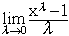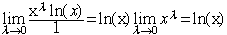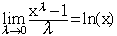I am wassim from Montreal ( student) and I have this problem in an econometrics course I need to know how to solve the: limits of (x ( to the power lamda) -1 )/LAMDA when lamda tends to zero ( the answer is that the functional form is ln x) and I still don't know how using hopital rule leads to this answer. Hi Wassim, As lamda tends to zero, xlamda tends to 1 and hence the numerator and denominator both tend to zero. Thus this problem is a candidate for l'hopital's rule.My assumption is that your difficulty is differentiating xlamda with respect to lamda. If you don't recall how to differentiate this form ( a constant raised to a variable power) you can proceed as follows. Let y = xlamda and take the natural log of both sides to get ln(y) = ln(xlamda) = lamda ln(x). Now differentiate both sides with respect to lamda. y'/y = ln(x) and hence y' = y ln(x) = xlamda ln(x). Now apply l'hopital's rule to your limit and consider the new limitThusCheers, Harley Go to Math Central